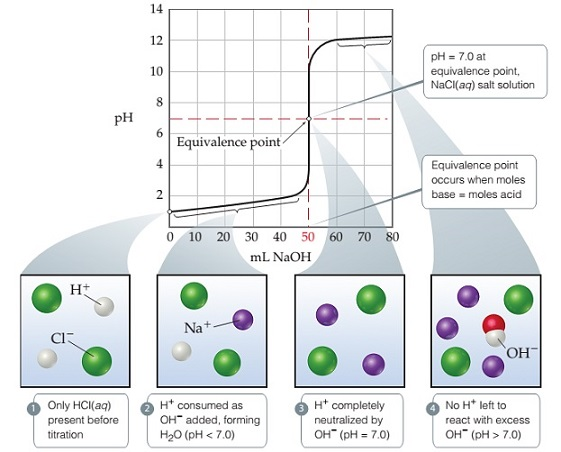# Problem: Titration of a strong acid with a strong base. The pH curve for titration of 50.0 mL of a 0.100 M solution of hydrochloric acid with a 0.100 M solution of NaOH(aq). For clarity, water molecules have been omitted from the molecular art.What volume of NaOH(aq) would be needed to reach the equivalence point if the concentration of the added base were 0.280 M?

###### FREE Expert Solution

We’re being asked to calculate the volume (in mL) of 0.280 M NaOH (strong base) required to reach the equivalence point in a titration of 50.00 mL of 0.100 M HCl (strong acid)

Recall that at the equivalence point of a titration:

Also, recall that moles = molarity × volume

This means:

$\overline{){\left(\mathbf{MV}\right)}_{{\mathbf{acid}}}{\mathbf{=}}{\left(\mathbf{MV}\right)}_{{\mathbf{base}}}}$

86% (184 ratings)###### Problem Details

Titration of a strong acid with a strong base. The pH curve for titration of 50.0 mL of a 0.100 M solution of hydrochloric acid with a 0.100 M solution of NaOH(aq). For clarity, water molecules have been omitted from the molecular art.What volume of NaOH(aq) would be needed to reach the equivalence point if the concentration of the added base were 0.280 M?